# (十六)ESP32: 双核执行和加速

## 计算加速

```start = micros();
//Run code
end = micros();
execTime = end - start;
```

## 全局变量

```int n  = {2,3,4,5,10,50,100,1000,2000,10000};
int nArraySize = 10;
```

```unsigned long start;
unsigned long end;
```

```SemaphoreHandle_t barrierSemaphore = xSemaphoreCreateCounting( 4, 0 );
```

```int bigArray, resultArray;
```

## FreeRTOS任务

```struct argsStruct {
int arrayStart;
int arrayEnd;
int n;
};
```

```argsStruct myArgs = *((argsStruct*)parameters);
```

```int product;
for (int i = myArgs.arrayStart; i < myArgs.arrayEnd; i++) {

product = 1;
for (int j = 0; j < myArgs.n; j++) {
product =  product * bigArray[i];
}
resultArray[i]=product;
//resultArray [i] = pow(bigArray[i], myArgs.n);
}
```

```void powerTask( void * parameters ) {

argsStruct  myArgs = *((argsStruct*)parameters);

int product;
for (int i = myArgs.arrayStart; i < myArgs.arrayEnd; i++) {

product = 1;
for (int j = 0; j < myArgs.n; j++) {
product =  product * bigArray[i];
}
resultArray[i]=product;
//resultArray [i] = pow(bigArray[i], myArgs.n);
}

xSemaphoreGive(barrierSemaphore);

}
```

## 设置功能

```Serial.begin(115200);
Serial.println();
```

```randomSeed(analogRead(0));
for (int i = 0; i < 10000; i++) {
bigArray[i] = random(1, 10);
}
```

```argsStruct oneTask = { 0 , 1000 , n[i] };

argsStruct twoTasks1 = { 0 , 1000 / 2 , n[i] };
argsStruct twoTasks2 = { 1000 / 2 , 1000 , n[i] };

argsStruct fourTasks1 = { 0 , 1000 / 4 , n[i] };
argsStruct fourTasks2 = { 1000 / 4 , 1000 / 4 * 2, n[i]};
argsStruct fourTasks3 = { 1000 / 4 * 2, 1000 / 4 * 3, n[i]};
argsStruct fourTasks4 = { 1000 / 4 * 3 , 1000, n[i]};
```

```Serial.println("");

start = micros();

10000,                   /* Stack size in words */
20,                      /* Priority of the task */
1);                      /* Core where the task should run */

xSemaphoreTake(barrierSemaphore, portMAX_DELAY);

end = micros();
Serial.print("Exec time: ");
Serial.print("Start: ");
Serial.println(start);
Serial.print("end: ");
Serial.println(end);
```

```int n = {2, 3, 4, 5, 10, 50, 100, 1000, 2000, 10000  };
int nArraySize = 10;

struct argsStruct {
int arrayStart;
int arrayEnd;
int n;
};

unsigned long start;
unsigned long end;

SemaphoreHandle_t barrierSemaphore = xSemaphoreCreateCounting( 4, 0 );

int bigArray, resultArray;

void setup() {

Serial.begin(115200);
Serial.println();

for (int i = 0; i < 10000; i++) {
bigArray[i] = random(1, 10);
}

for (int i = 0; i < nArraySize; i++) {

Serial.println("#############################");
Serial.print("Starting test for n= ");
Serial.println(n[i]);

argsStruct oneTask = { 0 , 1000 , n[i] };

argsStruct twoTasks1 = { 0 , 1000 / 2 , n[i] };
argsStruct twoTasks2 = { 1000 / 2 , 1000 , n[i] };

argsStruct fourTasks1 = { 0 , 1000 / 4 , n[i] };
argsStruct fourTasks2 = { 1000 / 4 , 1000 / 4 * 2,   n[i]};
argsStruct fourTasks3 = { 1000 / 4 * 2, 1000 / 4 * 3, n[i]};
argsStruct fourTasks4 = { 1000 / 4 * 3 , 1000,     n[i]};

Serial.println("");

start = micros();

10000,                   /* Stack size in words */
20,                      /* Priority of the task */
1);                      /* Core where the task should run */

xSemaphoreTake(barrierSemaphore, portMAX_DELAY);

end = micros();
Serial.print("Exec time: ");
Serial.print("Start: ");
Serial.println(start);
Serial.print("end: ");
Serial.println(end);

Serial.println("");

start = micros();

10000,                    /* Stack size in words */
20,                       /* Priority of the task */
0);                       /* Core where the task should run */

10000,                   /* Stack size in words */
20,                      /* Priority of the task */
1);                      /* Core where the task should run */

for (int i = 0; i < 2; i++) {
xSemaphoreTake(barrierSemaphore, portMAX_DELAY);
}

end = micros();
Serial.print("Exec time: ");
Serial.print("Start: ");
Serial.println(start);
Serial.print("end: ");
Serial.println(end);

Serial.println("");

start = micros();

10000,                    /* Stack size in words */
20,                       /* Priority of the task */
0);                       /* Core where the task should run */

10000,                    /* Stack size in words */
20,                       /* Priority of the task */
0);                       /* Core where the task should run */

10000,                    /* Stack size in words */
20,                       /* Priority of the task */
1);                       /* Core where the task should run */

10000,                    /* Stack size in words */
20,                       /* Priority of the task */
1);                       /* Core where the task should run */

for (int i = 0; i < 4; i++) {
xSemaphoreTake(barrierSemaphore, portMAX_DELAY);
}

end = micros();
Serial.print("Exec time: ");
Serial.print("Start: ");
Serial.println(start);
Serial.print("end: ");
Serial.println(end);

Serial.println();
Serial.println("------Results-------");

Serial.println("#############################");
Serial.println();
}

}

void loop() {

}

void powerTask( void * parameters ) {

argsStruct  myArgs = *((argsStruct*)parameters);

int product;
for (int i = myArgs.arrayStart; i < myArgs.arrayEnd; i++) {

product = 1;
for (int j = 0; j < myArgs.n; j++) {
product =  product * bigArray[i];
}

resultArray[i]=product;
//resultArray [i] = pow(bigArray[i], myArgs.n);
}

xSemaphoreGive(barrierSemaphore);

}
```

## 测试代码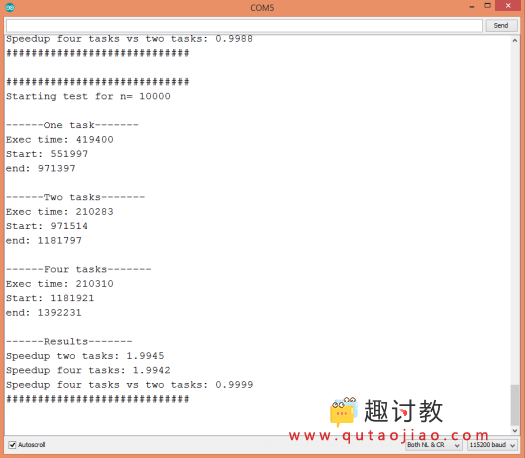## Amdahl定律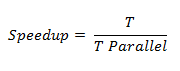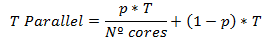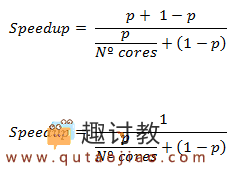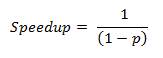## stm32核心板文件

2019-2-18 19:52:09

## (十七)ESP32：HTTP GET请求

2019-3-1 23:28:18

0 条回复 A文章作者 M管理员
暂无讨论，说说你的看法吧